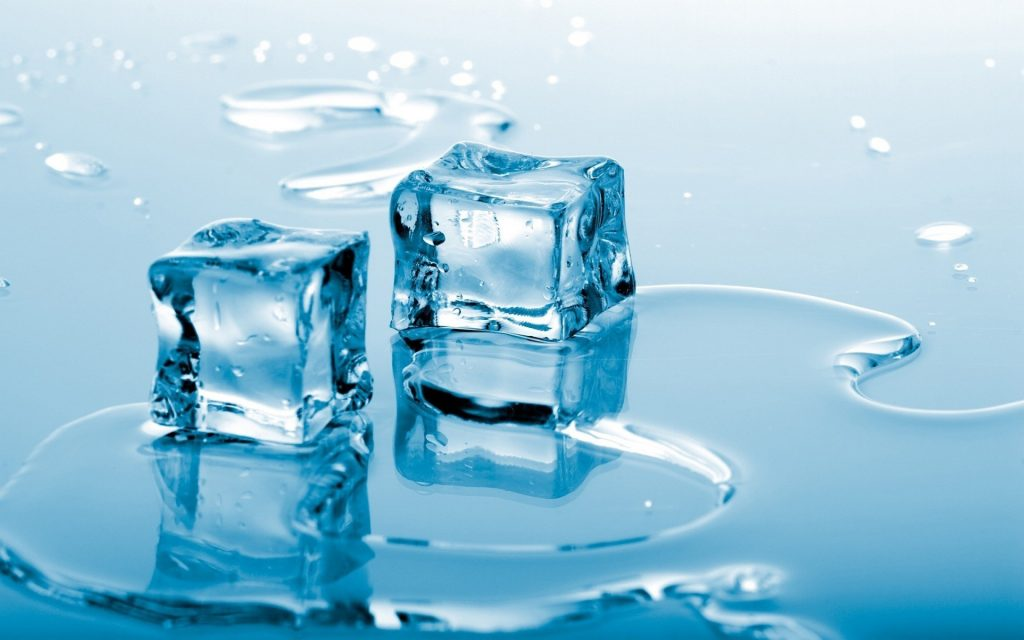E=MC2 must be one of the most well known equations. But what does it mean? What is E amd why is it equal to MC2 ?

In a Nutshell :   E=mc2 because mass and energy are two sides of the same coin.

Einstein’s famous equation is a mathematical way to express something called the equivalence of mass and energy. Although in everyday life we think of mass and energy as being very different things, physicists consider them to be different properties of the same thing.

In Einstein’s equation, the E stands for energy, m stands for mass and c stands for the speed of light. His equation says that mass and energy are interchangeable, and if you want to figure out how much energy is equivalent to a given amount of mass (or vice versa), you do the conversion by multiplying or dividing by the speed of light (c) squared. Often the speed of light is measured in miles per second, so that c2 = 186,282 x 186,282 = 34,700,983,524 m/s, which is a lot. But you can set the units in the equation to be what you want. If you set them so that c=1, then E=mc2 becomes E=mI2. The square of 1 is 1, so this is just the same as saying E=m or energy = mass.

When you sunbathe and heat up, you get heavier.

Einstein worked out his equation after he realized that an object becomes heavier when it gains energy (technically, it becomes more massive because weight and mass are not the same thing, but for everyday purposes we can say ‘heavier’). When you sunbathe and heat up, you get heavier. When a cube of water freezes into ice, it gets lighter. But the amounts involved are so minute that you can’t detect them. To work out how much mass you get from a given amount of energy, you rearrange Einstein’s equation. Instead of E=mc2, write it as M=e/c2. In other words you have to divide energy by the square of ‘c‘, the speed of light, which is a huge quantity, so you get a tiny increase in mass as the result (when you sunbathe).When you sunbathe and heat up, you get heavier. When a cube of water freezes into ice, it gets lighter.

The fact that things get heavier as they get faster is very important to the question of why nothing can travel faster than light

For instance, a charged-up battery weighs about 0.0000000001 grams more than a battery that has been discharged. If you heat a gold bar weighing a kilogram up by 10C (50°F) it gains about 0.000000000014g. There are other types of energy apart from heat and chemical energy. To make something move, you give it kinetic energy. Every time you throw a ball, the ball gains kinetic energy and gets a tiny bit heavier. When a bowler in cricket bowls at 160 kph (100 mph) – which is not very often but its a nice round number – the ball gets 0.000000000002 grams heavier. The fact that things get heavier as they get faster is very important to the question of why nothing can travel faster than light.

This relationship also works the other way round: mass is equivalent to energy, so when something gets less massive, it releases energy. We can say that the mass has been converted to energy. And because you can work out how much energy by multiplying the mass by the speed of light squared, you can see that even a tiny amount of mass can be converted into a lot of energy. This is the basis of the nuclear bomb, and also of the nuclear fusion reaction that powers the sun. The energy content of just one kilogram of matter would be enough to lift the population of the Earth into space.

As a final thought : If you were converted entirely into energy, you would explode more powerfully than 30 nuclear bombs!